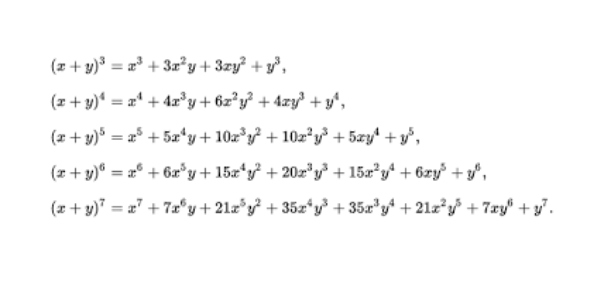# Additional Maths Binomial Probability Quiz

10 Questions | Attempts: 245
ShareSettingsBinomial probability refers to the probability that a binomial experiment result in exactly x successes. Meteorologists as well as economic conditions due to the economic changes and nature of the changes can apply this in real life situations such as weather forecasting. Additional Maths Binomial Probability Quiz will guide your understanding of this. Success.

• 1.
Lotte takes a test. Each question in the test has 4 answers. There are 10 questions in the test. She chooses one of the answers (a)-(d) for each question at random.The probability that she gets all 10 wrong is:
• A.

7.5

• B.

0.75

• C.

0.0563

• D.
• 2.
Sruthi takes a test. Each question in the test has 4 answers. There are 10 questions in the test. She chooses one of the answers (a)-(d) for each question at random.The probability that she gets exactly 9 wrong is:
• A.
• B.

0.188

• C.

0.0188

• D.

None of these

• 3.
Neha takes a test. Each question in the test has 4 answers. There are 10 questions in the test. She chooses one of the answers (a)-(d) for each question at random.The probability that she gets exactly 5 wrong is:
• A.

0.188

• B.

0.0584

• C.
• D.

None of these

• 4.
Milly takes a test. Each question in the test has 4 answers. There are 10 questions in the test. She chooses one of the answers (a)-(d) for each question at random.The probability that she gets at least 9 wrong is:
• A.

0.244

• B.

0.188

• C.

0.999999046

• D.

None of these

• 5.
Karishma takes a test. Each question in the test has 4 answers. There are 10 questions in the test. She chooses one of the answers (a)-(d) for each question at random.The probability that she gets at least 9 correct is:
• A.

0.756

• B.
• C.

0.812

• D.

None of these

• 6.
I choose 6 glasses at random from a large batch of glasses, 20% of which are faulty. Find the probability that, of the 6 chosen, none are faulty.
• A.

0.262

• B.

0.8

• C.
• D.
• 7.
I choose 6 glasses at random from a large batch of glasses, 20% of which are faulty. Find the probability that, of the 6 chosen, exactly 2 are faulty.
• A.

0.0164

• B.

0.0154

• C.
• D.

0.246

• 8.
I choose 6 glasses at random from a large batch of glasses, 20% of which are faulty. Find the probability that, of the 6 chosen, exactly 4 are faulty.
• A.

0.0154

• B.
• C.
• D.

0.4096

• 9.
I choose 6 glasses at random from a large batch of glasses, 20% of which are faulty. Find the probability that, of the 6 chosen, at least 1 is faulty.
• A.

0.738

• B.

0.393

• C.

0.2

• D.

0.998

• 10.
I choose 6 glasses at random from a large batch of glasses, 20% of which are faulty. Find the probability that, of the 6 chosen, at least 2 are good.
• A.

0.655

• B.
• C.

0.998

• D.

0.345

## Related TopicsBack to top
×

Wait!
Here's an interesting quiz for you.